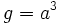# Complemented central factor not implies direct factor

This article gives the statement and possibly, proof, of a non-implication relation between two subgroup properties. That is, it states that every subgroup satisfying the first subgroup property (i.e., complemented central factor) need not satisfy the second subgroup property (i.e., direct factor)
View a complete list of subgroup property non-implications | View a complete list of subgroup property implications
Get more facts about complemented central factor|Get more facts about direct factor
EXPLORE EXAMPLES YOURSELF: View examples of subgroups satisfying property complemented central factor but not direct factor|View examples of subgroups satisfying property complemented central factor and direct factor

## Statement

### Statement with symbols

It is possible to have a group$G$ and a subgroup$H$ of$G$, such that$H$ is a complemented central factor of$G$ (i.e.,$HC_G(H) = G$ and there is a subgroup$K$ of$G$ such that$HK = G$ and$H \cap K$ is trivial) but$H$ is not a direct factor of$G$.

## Proof

### Example of the central product of the dihedral group of order eight and the cyclic group of order four

Further information: Central product of D8 and Z4

Consider the group obtained as the central product of dihedral group:D8 and cyclic group:Z4, sharing a common subgroup of order two. The presentation is:$G := \langle a,x,y \mid a^4 = x^2 = e, y^2 = a^2, xax = a^{-1}, xy = yx, ay = ya \rangle$.

Let$H = \langle a,x \rangle$ be the central factor that is the dihedral group of order eight.

Then we have:

•$H$ is a central factor of$G$: This is by construction;$G$ is the central product of$H$ and the four-element subgroup$\langle a \rangle$.
•$H$ is complemented in$G$: The subgroup$\langle ay \rangle$ is a subgroup of order two that is a complement to$H$ in$G$.
•$H$ is not a direct factor of$G$: There is no element of order two in$G$ outside$H$ that commutes with every element of$H$. To see this, note that any element of$G$ outside$H$ is of the form$gy,g \in H$. Its square is$g^2y^2 = g^2a^2$, since$y$ commutes with every element of$H$. Thus, the only way the element can have order two is if$g^2 = a^2$, forcing$g = a$ or$g = a^3$. However, in neither of these cases does$gy$ centralize$H$.